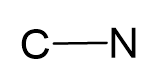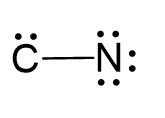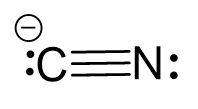## Examples

Connect the atoms together and count the number of electrons by adding 1 since there is a negative charge:#e = 4 + 5 + 1 = 10

Two electrons are used to make the covalent bond, therefore, the remaining 8 go to nitrogen as 3 lone pairs, and two to the carbon as a lone pair:Use two lone pairs from the nitrogen to make a triple bond with the carbon which is left with a lone pair and therefore, a negative charge:Two atoms have one atom connected and therefore, both geometries are linear. Additionally, notice that the hybridization state for the C and N is sp which implies a linear geometry.

Steric number 2 corresponds to sp-hybridization where the bond angles are 180o. Both atoms are sp-hybridized.